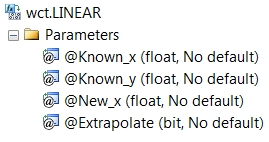# SQL Server linear interpolation with extrapolation

LINEAR

Updated: 20 November 2013

Note: This documentation is for the SQL2008 (and later) version of this XLeratorDB function, it is not compatible with SQL Server 2005.

Use the aggregate function LINEAR to calculate the straight-line interpolated value of y given x in the array (x, y). LINEAR is not sensitive to the order of the array (x, y). LINEAR supports extrapolation when the new x-value is outside the bounds of the supplied x-values or permits you to return the y-value associated with maximum x-value or the y-value associated with the minimum x-value when the new x-value is greater than the maximum x-value or less than the minimum x-value.
SyntaxArguments
@Known_x
the x-values to be used in the interpolation calculation. @Known_x must be of the type float or of a type that implicitly converts to float.
@Known_y
the y-values to be used in the interpolation calculation. @Known_y must be of the type float or of a type that implicitly converts to float.
@New_x
the new x-value for which you want LINEAR to calculate the y-value. @New_x must be of the type float or of a type that implicitly converts to float.
@Extrapolate
a bit value that identifies whether to return the boundary value or the extrapolated value when @New_x is outside the boundary. @Extrapolate must be of a type bit or of a type that implicitly converts to bit.
Return Types
float
Remarks
·         For cubic spline interpolation use the SPLINE function.
·         For polynomial interpolation, use the POLYINTERP function.
·         For monotonic spline interpolation use the MONOSPLINE function.
·         For bilinear interpolation use the INTERP2 function.
·         If @New_x is less than the smallest x-value and @Extrapolate is TRUE, then an extrapolated value is returned, otherwise the y-value paired with the smallest x-value is returned.
·         If @New_x is greater than the largest x-value and @Extrapolate is TRUE, then an extrapolated value is returned, otherwise the y-value paired with the largest x-value is returned.
·         If @Extrapolate is NULL then @Extrapolate is equal to FALSE.
·         If an x-y pair contains a NULL, then the pair is not used in the interpolation calculation.
·         If @New_x = @Known_x then @Known_y is returned.
·         @New_x must remain invariant for the GROUP.
·         @Extrapolate must remain invariant for the GROUP.
·         The number of rows passed into the function is less than 2 then a NULL is returned.
·         If @New_x is NULL then a NULL is returned.
Examples
Given the following x- and y-values, we want to calculate the y-value for x = 0.75
SELECT wct.LINEAR(x,y,0.75,NULL) as LINEAR
FROM (VALUES
(0.5,0.479426),
(1,0.841471),
(1.5,0.997495),
(2,0.909297),
(2.5,0.598472),
(3,0.14112),
(3.5,-0.350783),
(4,-0.756802)
) n(x,y)

This produces the following result.
LINEAR
----------------------
0.6604485

In this example we calculate the interpolated values with x-values below the minimum and above the maximum with @Extrapolate set to FALSE.
WITH mycte AS (
SELECT *
FROM (VALUES
(0.5,0.479426),
(1,0.841471),
(1.5,0.997495),
(2,0.909297),
(2.5,0.598472),
(3,0.14112),
(3.5,-0.350783),
(4,-0.756802)
) n(x,y)
)
SELECT m.x
,wct.LINEAR(mycte.x, mycte.y,m.x,'False') as LINEAR
FROM (VALUES
(-0.5)
,(0)
,(4.5)
,(5.0)
)m(x), mycte
GROUP BY m.x

This produces the following result.
x                 LINEAR
---------------------- ----------------------
-0.5               0.479426
0               0.479426
4.5              -0.756802
5              -0.756802

Using the same data, but setting @Extrapolate to TRUE.
WITH mycte AS (
SELECT *
FROM (VALUES
(0.5,0.479426),
(1,0.841471),
(1.5,0.997495),
(2,0.909297),
(2.5,0.598472),
(3,0.14112),
(3.5,-0.350783),
(4,-0.756802)
) n(x,y)
)
SELECT m.x
,wct.LINEAR(mycte.x, mycte.y,m.x,'True') as LINEAR
FROM (VALUES
(-0.5)
,(0)
,(4.5)
,(5.0)
)m(x), mycte
GROUP BY m.x

This produces the following result.
x                 LINEAR
---------------------- ----------------------
-0.5              -0.244664
0               0.117381
4.5              -1.162821
5               -1.56884

In this example, we will interpolate using dates.

SELECT wct.LINEAR(
CAST(CAST(x as datetime) as float)
,y
,CAST(CAST('2012-Oct-31' as datetime) as float)
,NULL) as LINEAR
FROM (VALUES
('2012-Apr-30',0.0028),
('2013-Apr-30',0.0056),
('2014-Apr-30',0.0085),
('2016-Apr-30',0.0164),
('2018-Apr-30',0.0235),
('2021-Apr-30',0.0299),
('2031-Apr-30',0.0382),
('2041-Apr-30',0.0406)
) m(x, y)

This produces the following result.
LINEAR
----------------------
0.00421150684931507

### SupportCopyright 2008-2021 Westclintech LLC         Privacy Policy        Terms of Service